# An Equivalent Circuit Model Reflecting the Current Dependency of Power Inductors and Evolving Simulations of Power Supply Circuits

Power inductors are one of the main components that constitute a power supply circuit. In a DC-DC converter, their effect is to suppress an alternating current, thereby smoothing out the electric current. However, they have dependency on the current; that is, their inductance values vary with DC superposition, which makes it hard for design engineers to choose right components or optimize their design. In response to customers’ request for more accurate and easier design environment, Murata has developed and started to distribute an equivalent circuit model that has reflected the current dependency of power inductors.　Presented here is an outline of the product together with application examples to simulations.

### What Is a Power Inductor?

Inductors may be broadly classified into two groups based on their applications: inductors for power lines and those for signal lines. Power inductors are components belonging to the first category, and they are used to smooth out an electric current in power supply circuits, such as a DC-DC converter. Murata offers two types of power inductors: a winding type and a monolithic type. Both of them use ferrites for a magnetic body (Fig. 1). Ferrites are ceramic compounds whose main constituent is iron oxide, and have a property to store magnetic energy in a highly dense manner. This property gives ferrite inductors poor permeability for the alternating portion of electric current, thereby exhibiting a great effect for suppressing noise or smoothing out the electric current. However, ferrites also exhibit magnetic saturation and magnetic loss.

Magnetic saturation is a state that an increase in externally applied magnetic field is no longer able to increase the magnetic flux density, thereby causing its magnetic energy to level off. The magnetic saturation of ferrites affects the DC superposition characteristics of power inductors. Figure 2 shows a measurement example of the DC superposition characteristics evaluated at 1MHz. As the DC current increases, the value of inductance shows a tendency to decline.

In the meantime, magnetic loss is a phenomenon where magnetic energy accumulated in ferrites is transformed into heat energy and is lost. Examples include hysteresis loss and eddy-current loss. When an electric current with a large amplitude flows in a power supply circuit, the magnetic loss in power inductors increases, thereby reducing the efficiency of the circuit. This suggests that it will be important to reflect the current dependency of power inductors in designing power supply circuits.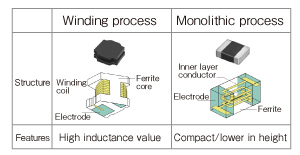Fig. 1 Types of Power Inductors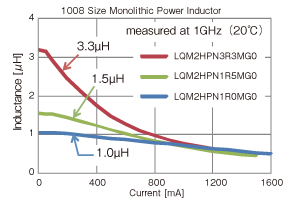Fig. 2 An Example Measurement of the DC Superposition Characteristics

### Present Situation and Challenges in Designing Power Supply Circuits

Normal operation of electronic devices, such as mobile phones and laptop computers, requires a stable power supply circuit that has little fluctuation of voltage. Among others, DC-DC converters have changed from a traditional linear type to a switching type, achieving a substantial reduction in size and weight, and a remarkable improvement of efficiency. Recently however, while IC driving voltage has been becoming smaller, the electric current that flows in circuits has become larger, requiring the adaptation for the larger current and further miniaturization of power inductors. Because efficiency as well as miniaturization is considered important in power supply circuits, it requires the selection of components and the optimization of design that satisfy the desired efficiency. Figure 3 plots the relationship between the efficiency of an experimental DC-DC converter unit and the volume of power inductors to be built in. The output current was set to 200mA to measure the efficiency. Power inductors are monolithic type (LQM series, all 18 product types), and all other components are the same. In this figure, you see a trade-off between the efficiency and the volume of power inductors. Design engineers must take into account this kind of relationship to select the components and optimize their design.Fig. 3 Relation Between the Efficiency and the Volume of the LQM Series (All 18 Product Types)

Optimization of design is an area where the application of simulations is anticipated, but in reality, trial and error experiments are used in quite a few power supply circuits. This is because simulations that take into account the current dependency of power inductors are difficult to accomplish, making it impossible to produce calculation results accurate enough to conduct the efficiency optimization. Figure 4 shows an example of traditional models provided by download at the Murata website so far. Traditional models, composed of only passive elements of LCR, are manufactured based on their characteristics when there is no DC superposition. Figure 5 compares the measured value of the DC superposition characteristics with their simulated value. The value of inductance declines as the electric current increases in the measured value, whereas the inductance value does not change in the simulated value. As this example suggests, the models that reflect no current dependency do not produce sufficiently accurate calculation results in simulations of the efficiency and response characteristics of a power supply circuit. Meanwhile, compatibility of speed and quality is strongly desired at the site of design work. To satisfy both, it is important to improve the accuracy of simulations to a practically acceptable level, and reduce the number of re-designing work or the number of prototypes constructed. In the design of a power supply circuit, because power inductors have a great impact on the performance of an entire circuit, it has been a challenge to develop an equivalent circuit model that reflects current dependency.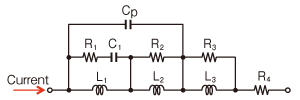Fig. 4 An Example of the Traditional Model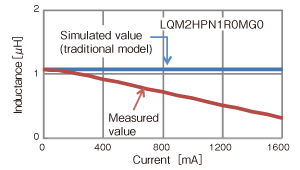Fig. 5 Comparison of the Measured Value and the Simulated Value of the DC Superposition Characteristics

### An Equivalent Circuit Model That Reflects Current Dependency

Figure 6 outlines an equivalent circuit model that we propose this time. On the left is a basic circuit that reflects the circuit characteristics when a direct current has no superposition. The addition of inductive and capacitive circuits to the main resonant circuit helps correct the frequency characteristics of AC impedance (or Q factor). When there is a DC superposition, the characteristics, such as inductance, change. This effect corresponds to changing L and R in the main resonant circuit and the inductive circuit. On the right is an equivalent circuit model that can reflect the dependency of L and R due to the current. It consists of the basic circuit and an additional voltage source model. The voltage source model is an element that converts the effect of L and R varying with electric currents to voltages, and uses a current-controlled voltage source. The current-controlled voltage source is a component that can be used in the simulator. It has capability to detect the current flowing into a circuit and calculate the voltage using specified mathematical functions. In this example, because we use a function that sets the voltage to zero (V) when the current is zero (A), the circuit that has no voltage source model agrees with the equivalent circuit model when there is no DC superposition. When there is a DC superposition, the voltage of the voltage source model varies with the current flowing into the circuit, thereby allowing users to conduct highly accurate dynamic simulations.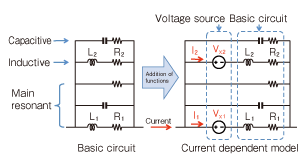Fig. 6 An Outline of the Proposed Equivalent Circuit Model

Note that, in switching DC-DC converters, because a current with a large amplitude flows though the power inductors, the magnetic loss in ferrites becomes larger. To take this loss into account, parameters of the equivalent circuit model are derived based on the measured values of the amplitude that corresponds to actual operation.

Figure 7 compares the simulated and measured values of the frequency characteristics of LQM2HPN1R0MG0 power inductor (1μH, rated current: 1600mA). You can confirm that, the inductance (left) and the AC impedance (right) exhibit a tendency to decline respectively as the DC current increases, whereas both simulated (solid line) and measured (dashed line) values exhibit good agreement. We have achieved the average error of less than 5% over the measured frequency range (500kHz-30MHz).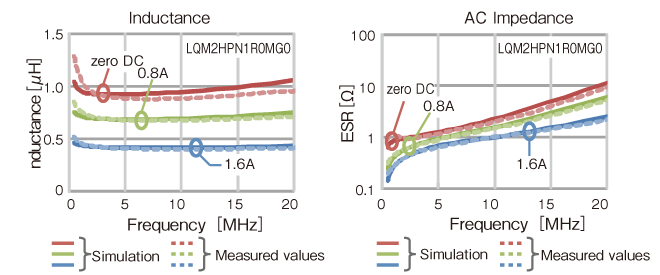Fig. 7 The Frequency Characteristics of Power Inductors

## Glossary

SPICE: An abbreviation of Simulation Program with Integrated Circuit Emphasis. A software program that simulates analog operation of electronic circuits.